# The Ultimate Skateboard Hardware Length Calculator

## Step 1: Measure Deck Thickness

Deck thickness is pretty straight forward. Figure out how thick your deck is, in inches.

as measured in inches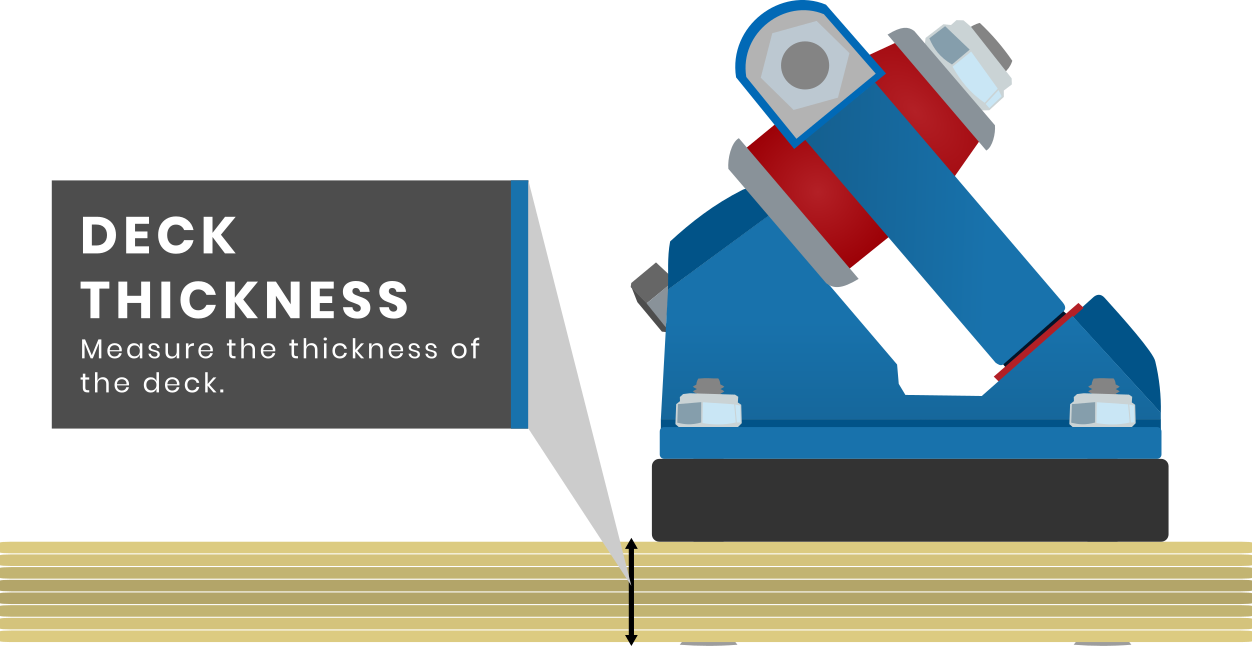## Step 2: Measure Riser Thickness

If you have a flat riser (no wedging), put the thickness of that riser here, in inches. Typical sizes are 1/8th (0.125 inches), 1/4th (0.25 in) and 1/2 inch (0.5 inches).

as measured in inches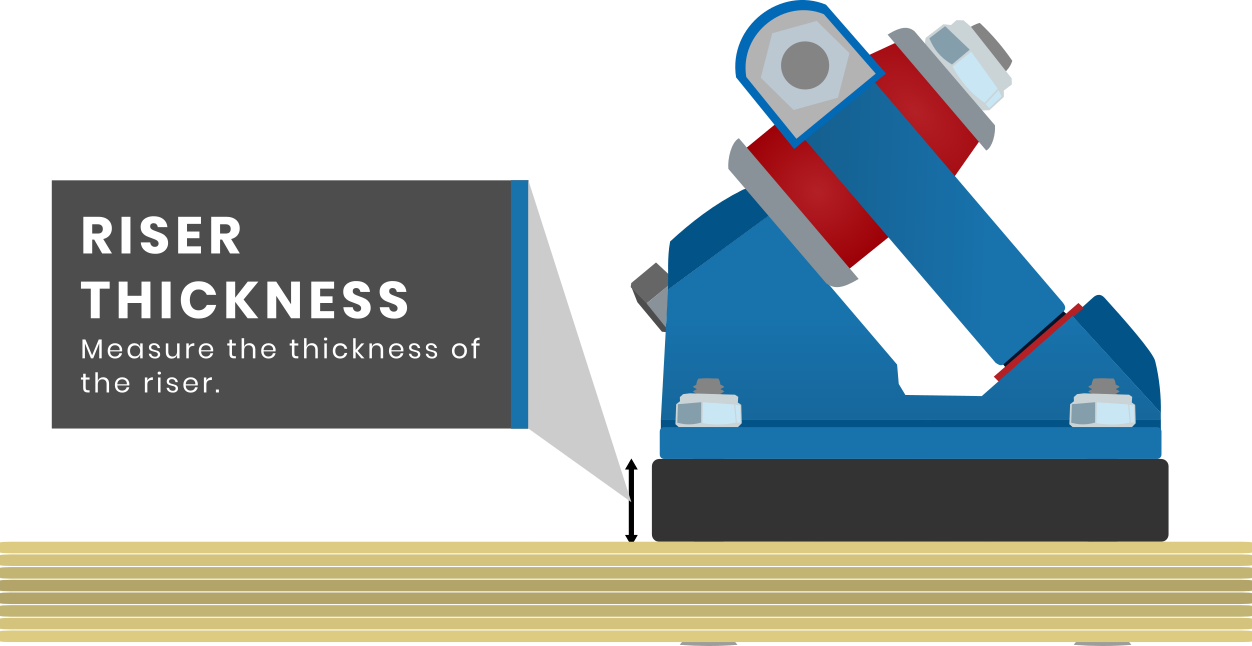## Step 3: Measure Wedge Thickness

If you are using a wedge riser, sometimes those risers have a rise and wedge. See photo for example. Measure this, in inches.

as measured in inches## Step 4: Wedge Degree

Here is where the fun begins. Put in your degree wedge for the calculator to do some trig.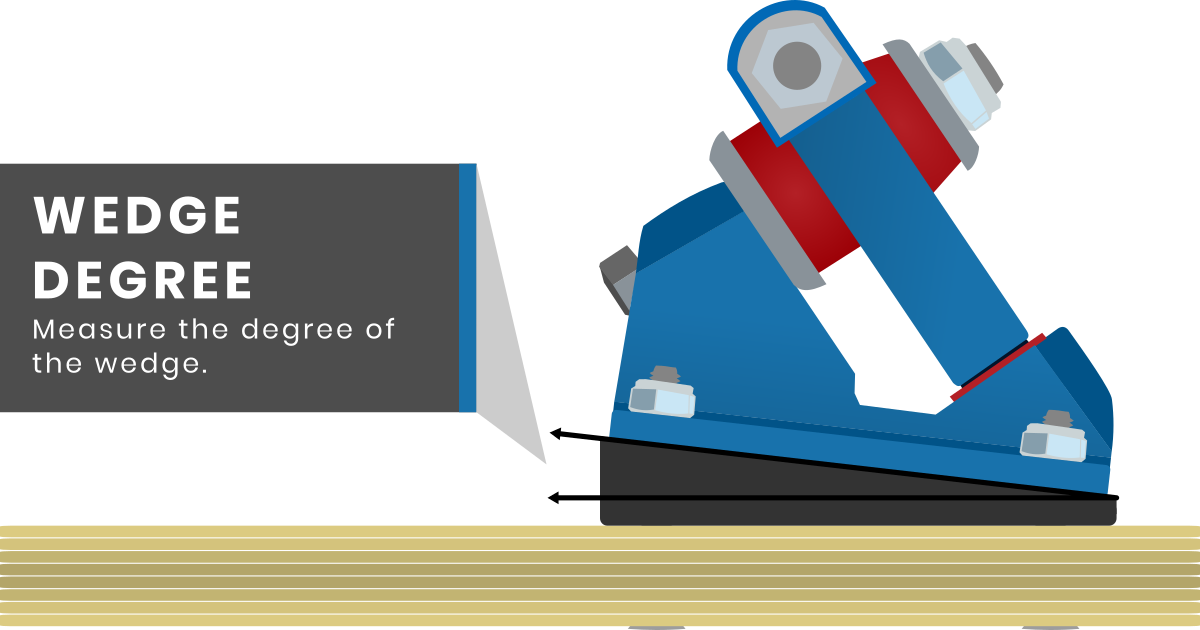## Step 5: Measure Baseplate Thickness

Not all baseplates are created equal. In the rare case you are using a flush mount deck, input 0 for this measurement.

as measured in inches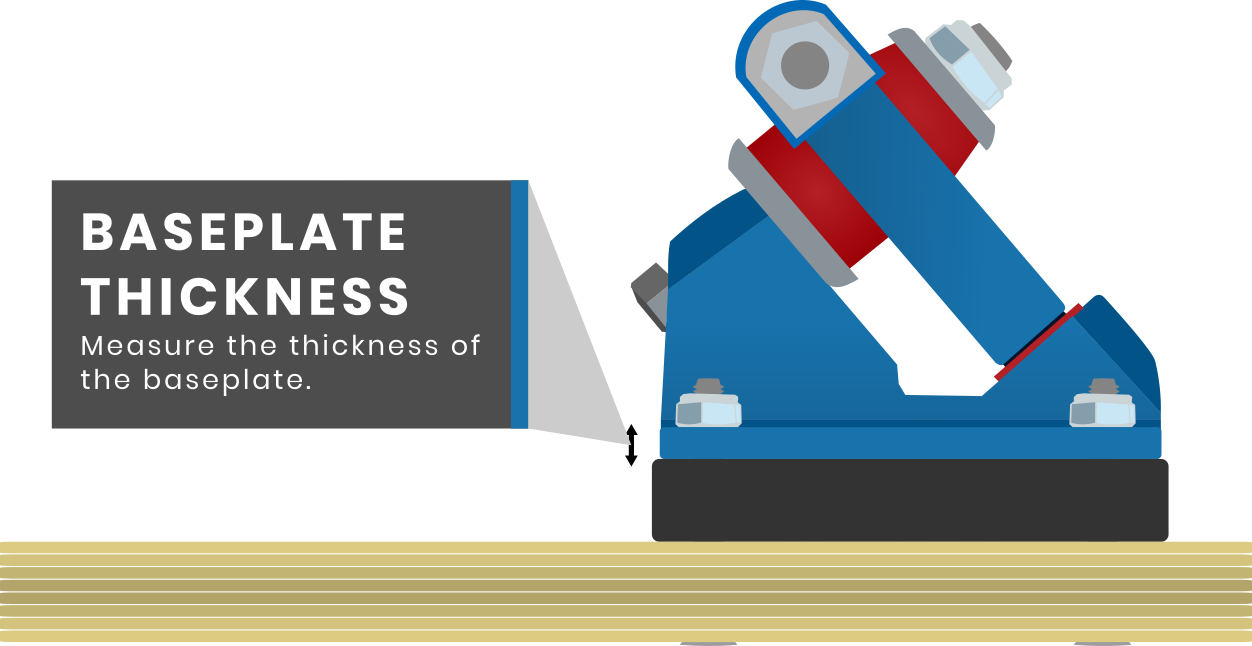## Hardware Results

SHORT SIDE LENGTH: LONG SIDE LENGTH:

### 1.00 in

Note that this is the absolute minimum length you need. You'll probably want to round up to the nearest quarter of an inch.

Click on the hardware length to be redirected to the correct hardware.

So Dr. Pham, What size of hardware do I need for my skateboard? Well that's simple, you need to add up the length of everything that's going to be mounted, your deck, risers, baseplate thickness, nut... but what if you have a wedge?

Oh boi, you done it now. Sit down while I skool you in 6th grade geometry. A wedge seen from the side is basically a right triangle. How do we find the length of the side of the triangle our hardware is going to go through?THE LAW OF SINES SON.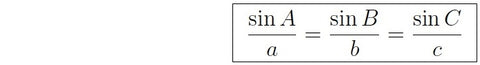Let's label our triangle.What do we know?

A is the angle of our wedge, easy.

C is 90 degrees, easy.

B? Here we need THE TRIANGLE POSTULATE, A+B+C=180 soooo... B=180-A-C or B=90-A

b is the truck mounting point on our board. We know how long that is, 2.5 inches, the standard length of old school skateboard mounting.

c doesn't matter.

a is what we're trying to find and with a little manipulation of the law of sines a = (b sin A) / sin B

Make the computer do all that rad math and you get the length of a, our longest side. Add it all up with the rest of the deck, risers, wedge, baseplate, nut, and you get the length you need.

But wait you say! What about the shorter hardware length?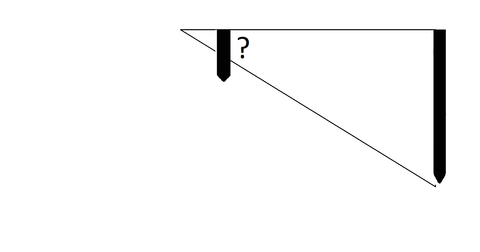That's the same problem with a smaller (and similar) triangle. In this case, b is the distance between the edge of the wedge and the center of the mounting point, a quarter inch.

And you said "when will we ever use this?" Now go apologize to your teachers.